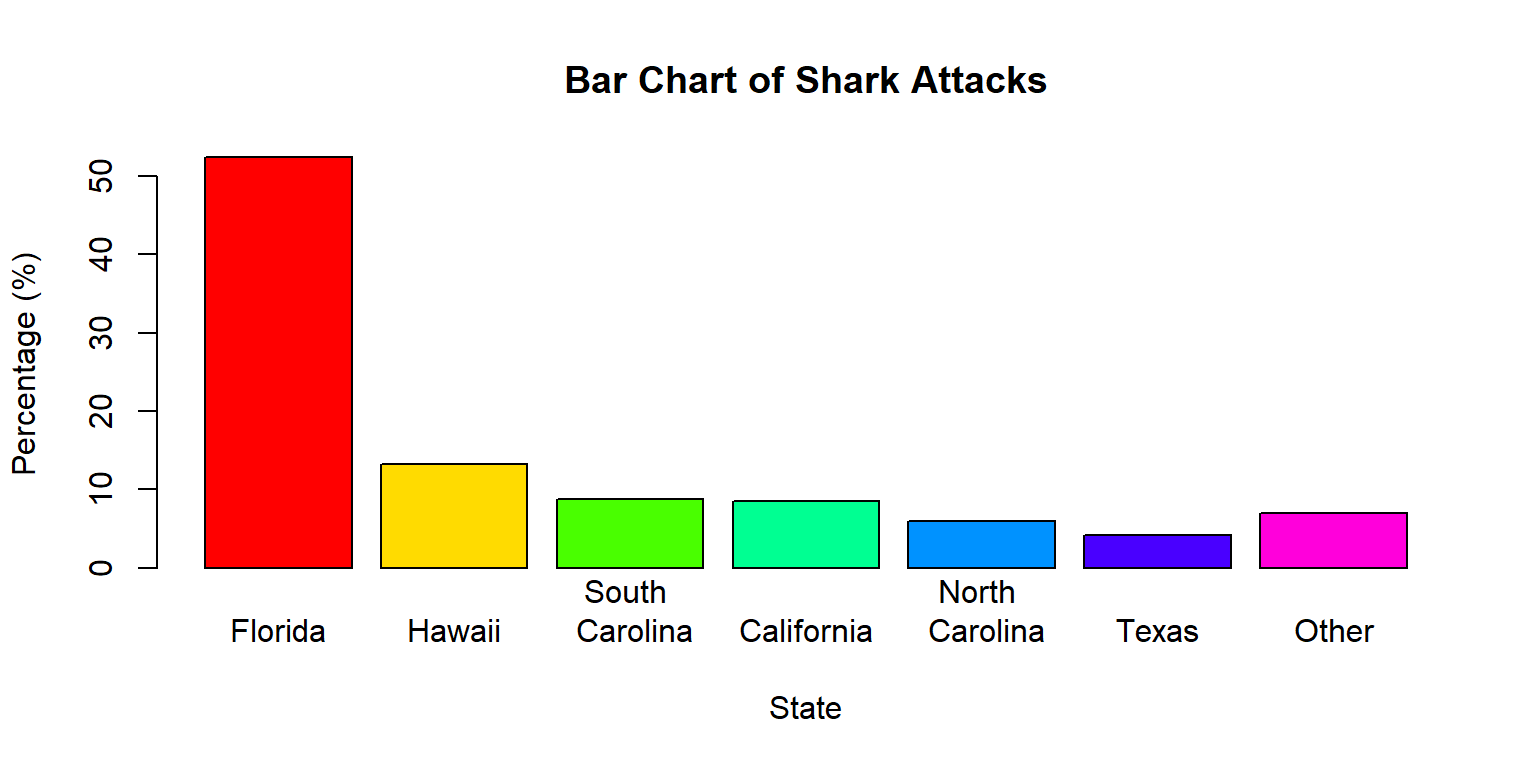# Chapter 2

## Example 3: Creating Pie Charts and Bar Charts

First, lets create the dataset from Example 3 from scratch:

State <- c("Florida", "Hawaii", "South Carolina", "California", "North Carolina",
"Texas", "Other")
Frequency <- c(203, 51, 34, 33, 23, 16, 27)

This is sufficient to construct a basic pie chart:

pie(Frequency, labels = State, col = rainbow(7), main = "Pie Chart of Shark Attacks")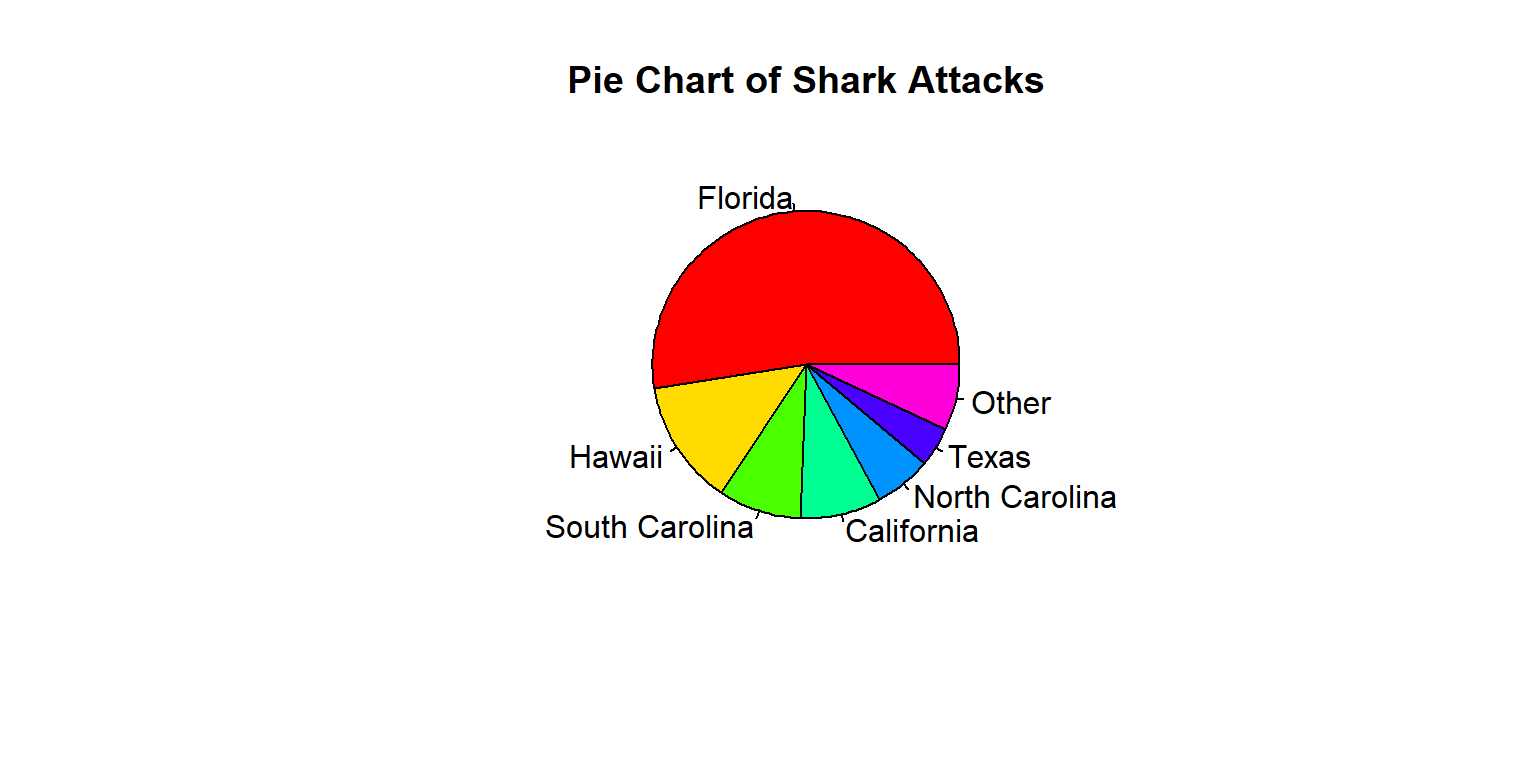You can get a basic barchart like this,

barplot(Frequency, names.arg = State, col = rainbow(7), main = "Bar Chart of Shark Attacks")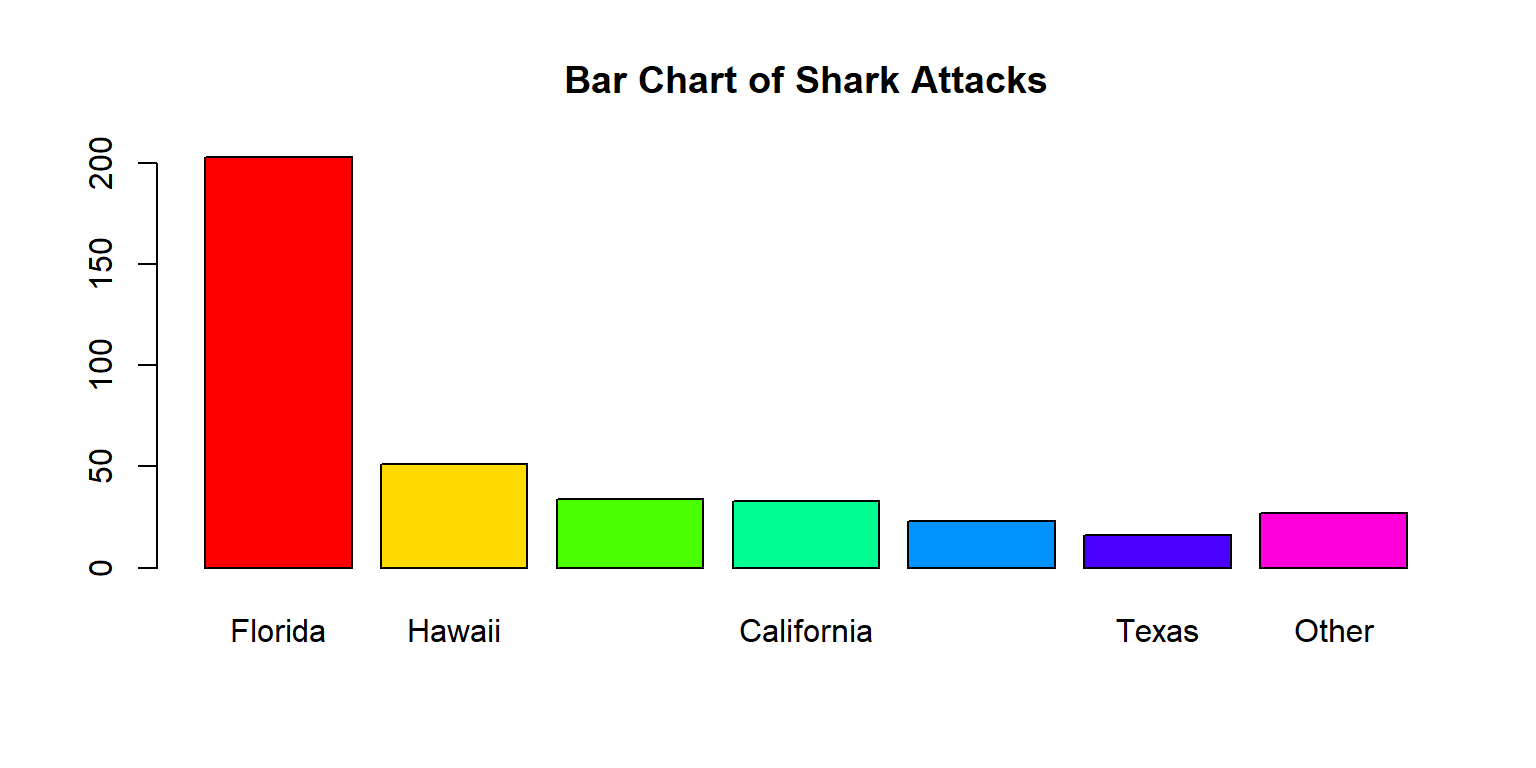but it is a good idea to add axis labels:

barplot(Frequency, names.arg = State, col = rainbow(7), main = "Bar Chart of Shark Attacks",
xlab = "State", ylab = "Frequency")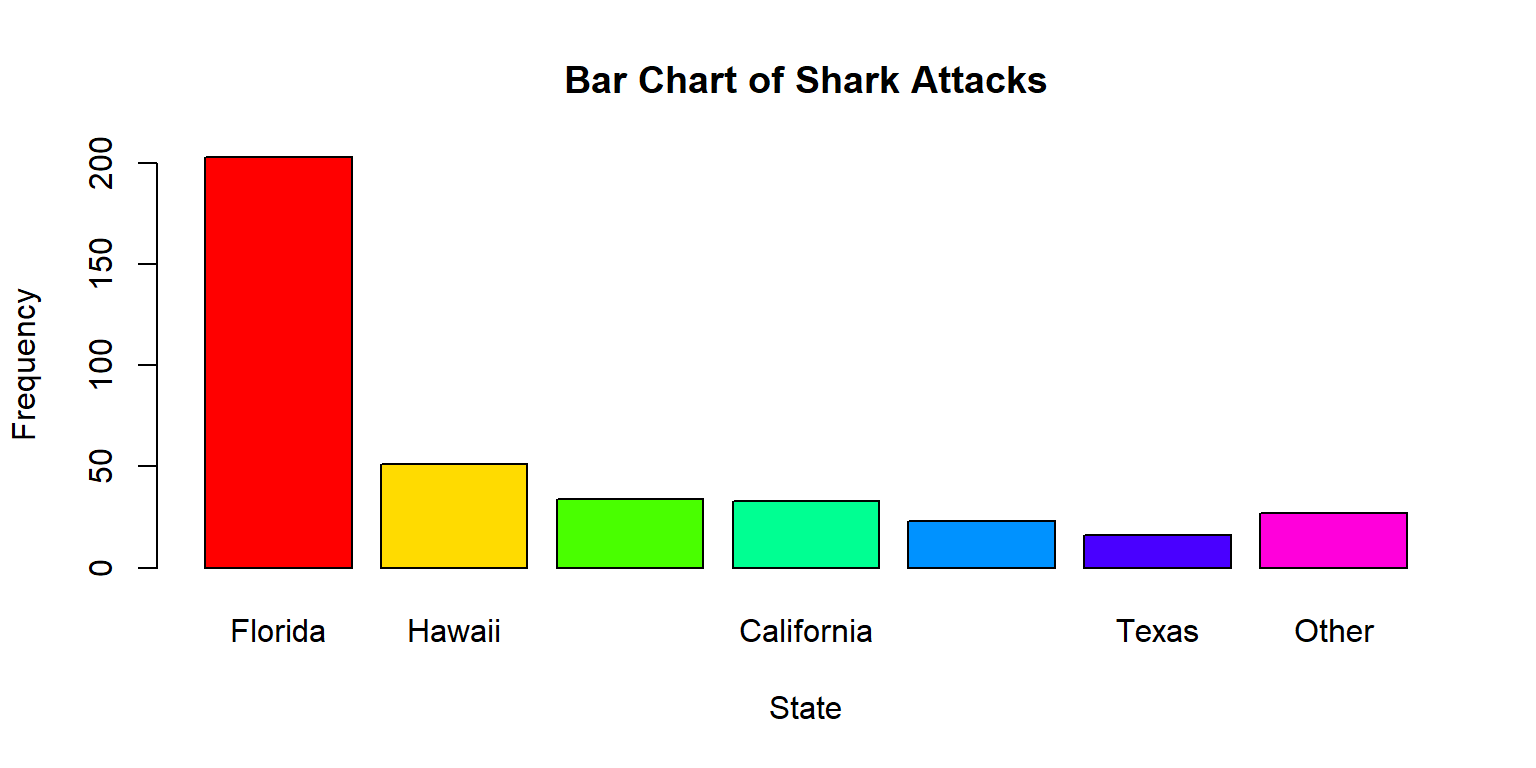Often, as in the graph above, the labels don’t all fit on the x-axis. Here are some workarounds.

Make the text smaller:

barplot(Frequency, names.arg = State, col = rainbow(7), cex.names = 0.8, main = "Bar Chart of Shark Attacks",
xlab = "State", ylab = "Frequency")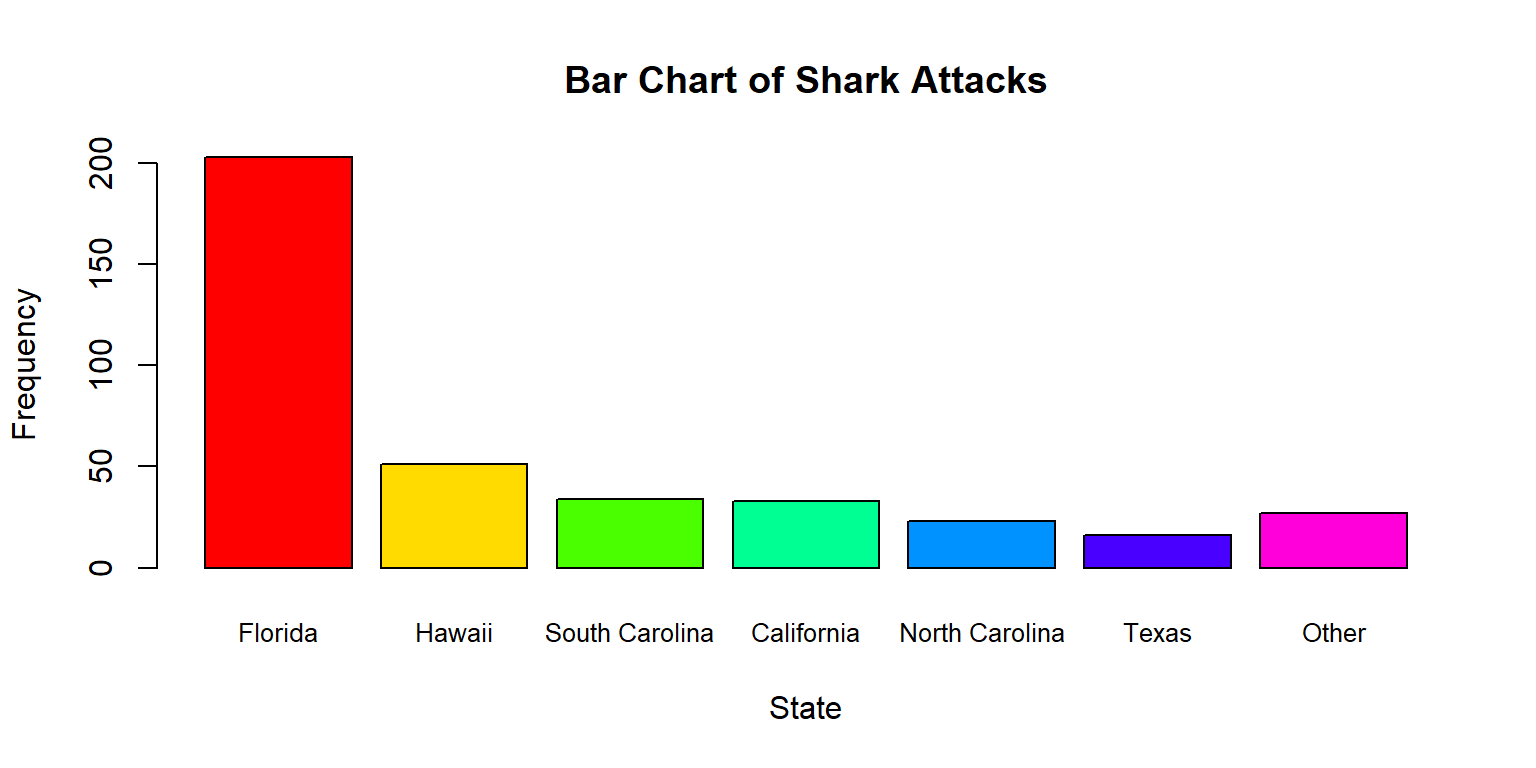Include a line break \n in some of the longer labels:

State <- c("Florida", "Hawaii", "South \n Carolina", "California", "North \n Carolina",
"Texas", "Other")
barplot(Frequency, names.arg = State, col = rainbow(7), main = "Bar Chart of Shark Attacks",
xlab = "State", ylab = "Frequency")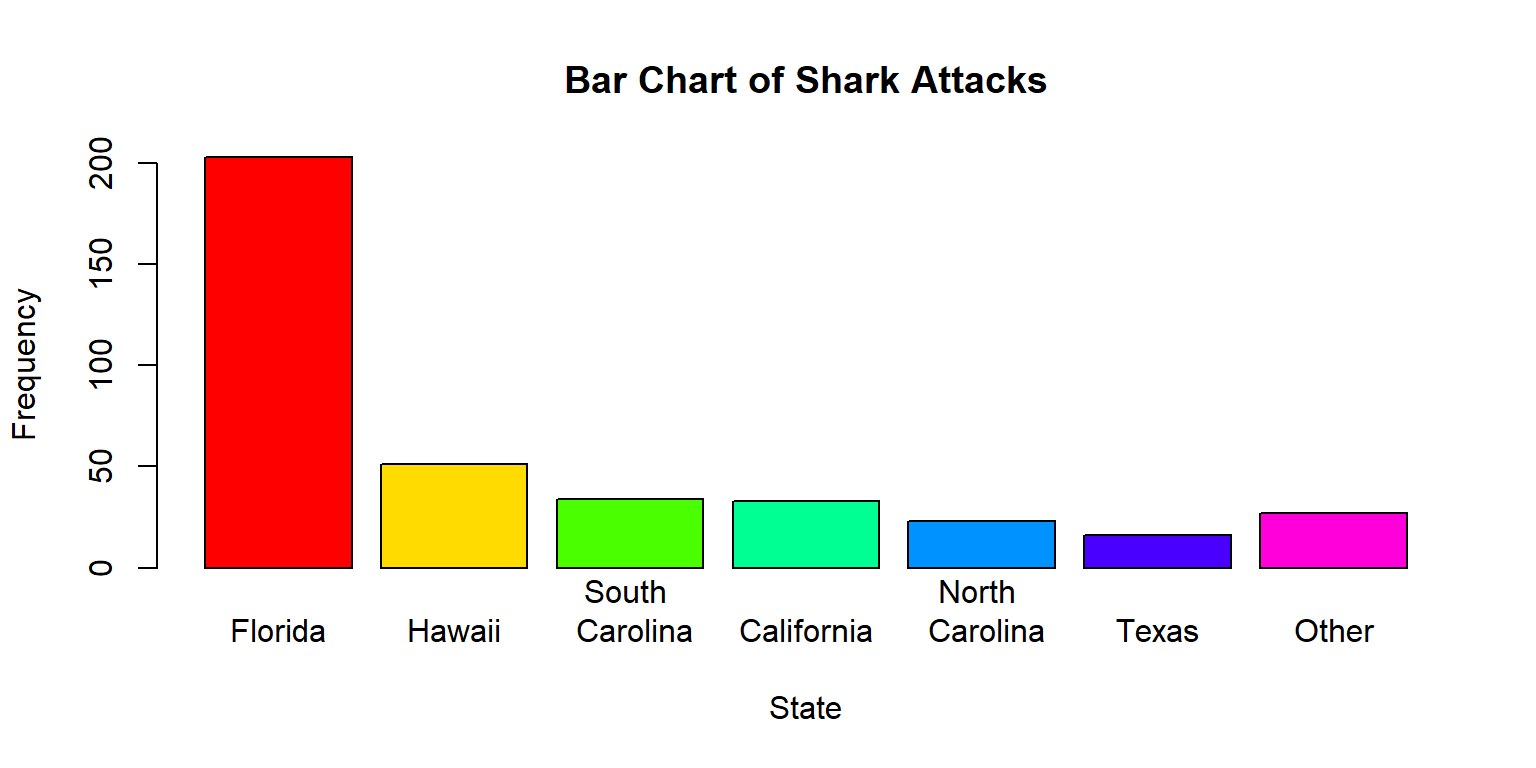Typically, a combination of these two will work.

This bar charts shows the counts of the categories on the y axis. In the book, we show percentages, which you can get like this:

Percentage <- 100 * Frequency/sum(Frequency)
barplot(Percentage, names.arg = State, col = rainbow(7), main = "Bar Chart of Shark Attacks",
xlab = "State", ylab = "Percentage (%)")